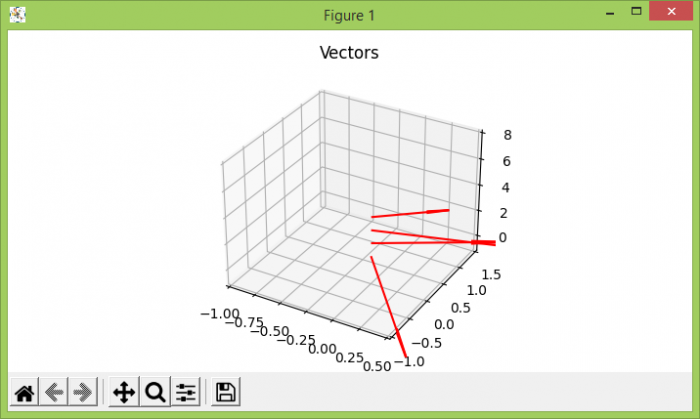# Putting arrowheads on vectors in Matplotlib's 3D plot

To draw arrow heads vectors in 3D matplotlb's plot, we can take the following steps −

• Create a 2D array, where x, y, z, u, v and are the coordinates of the arrow locations and direction components of arrow vectors.

• Using figure() method, create a new figure or activate an existing figure.

• Add an '~.axes.Axes' to the figure as part of a subplot arrangement, using add_subplot() method.

• Plot a 3D field of arrows, using quiver() method.

• Using ylim, xlim, zlim, limit the range of the axes.

• Set the title of the plot.

• To display the figure, use show() method.

## Example

import matplotlib.pyplot as plt
import numpy as np
plt.rcParams["figure.figsize"] = [7.00, 3.50]
plt.rcParams["figure.autolayout"] = True
soa = np.array([[0, 0, 1, 1, -2, 0], [0, 0, 2, 1, 1, 0], [0, 0, 3, 2, 1, 0], [0, 0, 4, 0.5, 0.7, 0]])
X, Y, Z, U, V, W = zip(*soa)
fig = plt.figure()
plt.show()